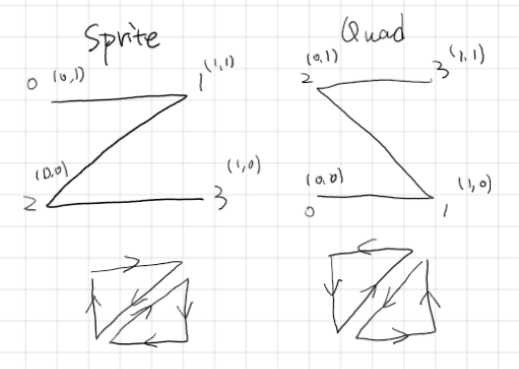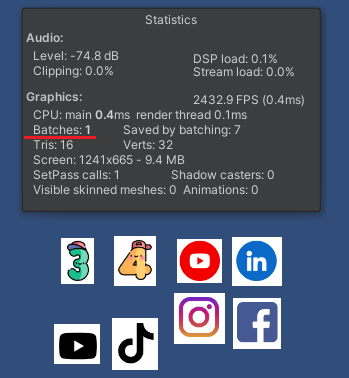# 资产特性

• 角色的精灵动画使用帧动画实现
• 每个角色的精灵帧动画在同一张Atlas中，并通过Sprite Mode “Multiple”和Sprite Editor来编辑并导入工程
• 每个角色的Atlas贴图格式相同且大小均为512X512

# 缩放与平移``````// 根据Sprite的尺寸和偏移计算Quad的缩放与平移关系
Pivot.x = sprite.rect.width / sprite.pixelsPerUnit;
Pivot.y = sprite.rect.height / sprite.pixelsPerUnit;
Pivot.z = ((sprite.rect.width / 2) - sprite.pivot.x) / sprite.pixelsPerUnit;
Pivot.w = ((sprite.rect.height / 2) - sprite.pivot.y) / sprite.pixelsPerUnit;
// 根据顶点顺序的不同重新映射正确的UV范围
newUV.x = sprite.uv.x - sprite.uv.x;
newUV.y = sprite.uv.y - sprite.uv.y;
newUV.z = sprite.uv.x;
newUV.w = sprite.uv.y;
``````

``````half4 pivot = UNITY_ACCESS_INSTANCED_PROP(Props, _Pivot);
// 生成缩放平移矩阵
half4x4 m;
m._11 = pivot.x; m._12 = 0; m._13 = 0; m._14 = pivot.z;
m._21 = 0; m._22 = pivot.y; m._23 = 0; m._24 = pivot.w;
m._31 = 0; m._32 = 0; m._33 = 1; m._34 = 0;
m._41 = 0; m._42 = 0; m._43 = 0; m._44 = 1;
float4 vertex = UnityFlipSprite(input.positionOS.xyz, _Flip);
// 生成UV的变换矩阵
half3x3 uvm;
half4 newUV = UNITY_ACCESS_INSTANCED_PROP(Props, _NewUV);
uvm._11 = newUV.x; uvm._12 = 0; uvm._13 = newUV.z;
uvm._21 = 0; uvm._22 = newUV.y; uvm._23 = newUV.w;
uvm._31 = 0; uvm._32 = 0; uvm._33 = 1;
half3 uv = half3(input.texcoord.x, input.texcoord.y, 1);
// 执行UV变换矩阵
uv = mul(uvm, uv);
VertexPositionInputs vertexInput;// = GetVertexPositionInputs(vertex.xyz);
// 执行顶点缩放平移变换
vertexInput.positionWS = mul(m, input.positionOS); // convert as Sprite
vertexInput.positionWS = TransformObjectToWorld(vertexInput.positionWS).xyz;
// ......
// 将变换后的UV赋值给片元的数据结构
output.uv.xy = uv.xy;
``````

# 着色器语法

• 启用贴图数组的必要宏：#pragma require 2darray
• 启用GPU Instancing的必要宏：#pragma multi_compile_instancing
• 声明贴图数组：Texture2DArray _TextureArray;
• 贴图采样：_Textures.Sample(my_point_clamp_sampler, float3(uv, _TextureIndex)
• 以及GPU Instancing必要的MaterialPropertyBlock中对应Buffer的声明

# Light Probe的支持

``````CBUFFER_START(UnityPerDraw)
// SH block feature
real4 unity_SHAr;
real4 unity_SHAg;
real4 unity_SHAb;
real4 unity_SHBr;
real4 unity_SHBg;
real4 unity_SHBb;
real4 unity_SHC;
CBUFFER_END
``````

``````// Linear + constant polynomial terms
float3 res = SHEvalLinearL0L1(output.normal.xyz, unity_SHAr, unity_SHAg, unity_SHAb);

res += SHEvalLinearL2(output.normal.xyz, unity_SHBr, unity_SHBg, unity_SHBb, unity_SHC);

#ifdef UNITY_COLORSPACE_GAMMA
res = LinearToSRGB(res);
#endif

output.vertexSH.xyz = max(half3(0, 0, 0), res);
``````

``````// Set Light Probe info
Vector3[] position = new Vector3 { transform.position };
SphericalHarmonicsL2[] lightProbes = new SphericalHarmonicsL2;
Vector4[] occlusionProbes = new Vector4;

// Manually get proper SH values
LightProbes.CalculateInterpolatedLightAndOcclusionProbes(position, lightProbes, occlusionProbes);
materialPropertyBlock.CopySHCoefficientArraysFrom(lightProbes);
materialPropertyBlock.CopyProbeOcclusionArrayFrom(occlusionProbes);

// We have to set light probe mode to "CustomProvided", so Unity will copy and use them into CBUFFER
meshRenderer.lightProbeUsage = LightProbeUsage.CustomProvided;
``````

# 动态Atlas

• Sprite Instancing会在运行时使用可能用到的最大贴图尺寸来创建初始的Texture2DArray（例1024×1024）
• 当不同尺寸的Sprite贴图加载进来的时候，我们通过Graphics.CopyTexture拷贝至Texture2dArray中，但拷贝的位置会根据算法自动分配其位于Texture2DArray中合适位置